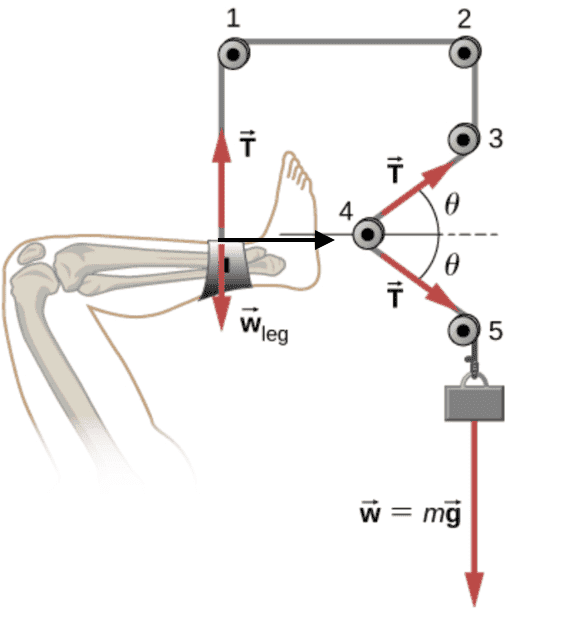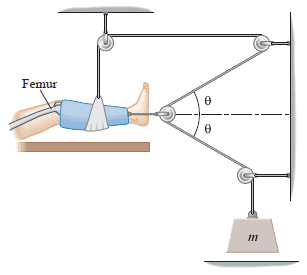# Leg suspended in a traction system

annamal
Homework Statement:
A leg is suspended in a traction system, as shown below. (a) Which pulley in the figure is used to calculate the force exerted on the foot? (b) What is the tension in the rope? Here T is the tension, wleg is the weight of the leg, and w is the weight of the load that provides the tension
Relevant Equations:
b) T = m*g. Why?
A leg is suspended in a traction system, as shown in the attached image. (a) Which pulley in the figure is used to calculate the force exerted on the foot? (b) What is the tension in the rope? Here T is the tension, wleg is the weight of the leg, and w is the weight of the load that provides the tension

b) I am not sure why T = m*g. Why wouldn't w = T*sin(theta) --> T = w/sin(theta)?

#### Attachments

•Screen Shot 2022-03-20 at 7.45.17 PM.png
24.9 KB · Views: 64

Homework Helper
Gold Member
2022 Award
Homework Statement:: A leg is suspended in a traction system, as shown below. (a) Which pulley in the figure is used to calculate the force exerted on the foot? (b) What is the tension in the rope? Here T is the tension, wleg is the weight of the leg, and w is the weight of the load that provides the tension
Relevant Equations:: b) T = m*g. Why?

A leg is suspended in a traction system, as shown in the attached image. (a) Which pulley in the figure is used to calculate the force exerted on the foot? (b) What is the tension in the rope? Here T is the tension, wleg is the weight of the leg, and w is the weight of the load that provides the tension

b) I am not sure why T = m*g. Why wouldn't w = T*sin(theta) --> T = w/sin(theta)?
Consider all the forces on that pulley. Clearly it cannot just float in the air like that.
Then consider the torques on that pulley. If the pulley's axle is frictionless, what can you deduce?

•Delta2 and Lnewqban
Homework Helper
Gold Member
A leg is suspended in a traction system, as shown in the attached image. (a) Which pulley in the figure is used to calculate the force exerted on the foot? (b) What is the tension in the rope? Here T is the tension, wleg is the weight of the leg, and w is the weight of the load that provides the tension
The vertical portion of the rope (ankle to pulley 1) is only keeping that leg bone horizontal.
There is a wire linking the foot and pulley 4, which is the one exerting the stretching force on that healing bone.
As neither the ankle nor the foot are moving, could we say that the system of x-y forces is in equilibrium?
What about the summation of all moments?

•nasu
annamal
I know this is late, but I figured out the solution to the problem. I am just wondering now whether the leg has a horizontal force as seen in image represented with the black arrow below:Homework Helper
Gold Member
2022 Award
I know this is late, but I figured out the solution to the problem. I am just wondering now whether the leg has a horizontal force as seen in image represented with the black arrow below:
View attachment 302759
If that is the only horizontal force on it, what will happen?

Homework Helper
Gold Member
I know this is late, but I figured out the solution to the problem. I am just wondering now whether the leg has a horizontal force as seen in image represented with the black arrow below:
View attachment 302759
What entity would exert a horizontal force? The leg is attached to a rope the pulls vertically up and then there is gravity from the Earth that pulls vertically down. The patient could be exerting a horizontal force with his/her muscles, but we are not told anything about it so we have to assume that it's not happening.

•Delta2
Homework Helper
Gold Member
2022 Award
The patient could be exerting a horizontal force with his/her muscles, but we are not told anything about it so we have to assume that it's not happening.
I think it is enough to assume that the diagrammed position is static.

•Delta2
Homework Helper
Gold Member
I think it is enough to assume that the diagrammed position is static.
Sure. You are looking at the RHS of Newton's second law whilst I am looking at the LHS.

•Delta2
Homework Helper
Gold Member
2022 Award
Sure. You are looking at the RHS of Newton's second law whilst I am looking at the LHS.
No, I am saying that stasis is implied, as is the verticality of the rope, so you do not need to assume there is no horizontal force from the leg.

Homework Helper
Gold Member
I know this is late, but I figured out the solution to the problem. I am just wondering now whether the leg has a horizontal force as seen in image represented with the black arrow below:
The leg's bone has a horizontal external force acting on it.

Copied from post #3:
There is a wire linking the foot and pulley 4, which is the one exerting the stretching force on that healing bone.

•Delta2
Homework Helper
Gold Member
I think the drawing in post #1 is misleading. The horizontal connection of pulley 4 to the leg is not at all obvious. Also not obvious is that pulley 4 is free but the rest of the pulleys are firmly attached to external fixtures. I found a more appropriately drawn picture shown below.If one draws an FBD of the foot and the lower leg that is in the blue cast as the system, it is clear that there is a horizontal force exerted to the left by the short rope attached to the pulley on the right. The system does not accelerate horizontally therefore there must be a horizontal force of equal magnitude to the left at the femur. The two horizontal forces at either end of the lower leg keep it under traction.

The original drawing can also be criticized for showing the thigh angled to the left of the vertical. It seems to me that if a horizontal force were pulling to the right, the thigh would rotate about the hip and be angled to the right of the horizontal unless the patient were actively opposing that force with his/her thigh muscles.

•annamal and Lnewqban
Homework Helper
Gold Member
2022 Award
The leg's bone has a horizontal external force acting on it.

Copied from post #3:
There is a wire linking the foot and pulley 4, which is the one exerting the stretching force on that healing bone.
How do you know this? It is not given in any post by the OP and is certainly not shown in the original diagram.
I found a more appropriately drawn picture shown below.
The leg is drawn very differently. In the diagram in post #1, there is no way that leg is under tension. If anything, it would require a force to the left to stop the leg moving to the right. It seems to me the two diagrams could represent different uses of the device.
That said, in the diagram in post #1, pulley 4 serves no useful purpose.
All very strange.

@annamal , where does that diagram come from?

Homework Helper
Gold Member
All very strange.
I am only guessing that the drawing here was created by an artist who didn't understand the importance of showing where and which of the pulleys are anchored. The artist was supervised by an editor who didn't understand statics or orthopaedics. Most importantly, the drawing was not examined carefully by the person who assigned it to @annamal. It happens.

annamal
How do you know this? It is not given in any post by the OP and is certainly not shown in the original diagram.

The leg is drawn very differently. In the diagram in post #1, there is no way that leg is under tension. If anything, it would require a force to the left to stop the leg moving to the right. It seems to me the two diagrams could represent different uses of the device.
That said, in the diagram in post #1, pulley 4 serves no useful purpose.
All very strange.

@annamal , where does that ditagram come from?
openstax volume 1 calculus physics. I figured it out. The drawing is not very good.

Homework Helper
Gold Member
The drawing is not very good.
I agree, it's not.

Homework Helper
Gold Member
2022 Award
openstax volume 1 calculus physics. I figured it out. The drawing is not very good.
Why am I not surprised? I recently began trawling through OpenStax, reporting errors. I don't think they'll be able to keep up, so I might create a page of corrections on Physics Forum.

Homework Helper
Gold Member
How do you know this? It is not given in any post by the OP and is certainly not shown in the original diagram.
Just personal experience from motorcyclist friends who have suffered leg fractures.
I believe it is called Hamilton-Russell traction system.
https://www.ncbi.nlm.nih.gov/pmc/articles/PMC7515792/

The stretching force is applied directly to the bone through metal pins inserted through it.
In the OP illustration, which is a poor representation of the actual apparatus, note that horizontal solid line joining the center of pulley 4 and the foot.

Homework Helper
Gold Member
2022 Award
note that horizontal solid line joining the center of pulley 4 and the foot.
That is quite clearly a construction line, indicating the horizontal. It continues out to the right, and shows no attempt to connect it to the foot.

Homework Helper
I believe it is called Hamilton-Russell traction system.
https://www.ncbi.nlm.nih.gov/pmc/articles/PMC7515792/

The stretching force is applied directly to the bone through metal pins inserted through it.
In the linked article, the diagram for Hamilton-Russel traction (which does indeed match this problem nicely) is described:
https://www.ncbi.nlm.nih.gov/pmc/articles/PMC7515792/ said:
A broad soft sling is placed under the knee that provides an upward force, which controls the posterior angulation of the distal fragment. Distal to the knee, skin traction (as described) is applied where the horizontal pull is on the tibia using cord, pulleys and weights. The mechanical forces are such that the horizontal pull is twice that of vertical pull which provides a resultant vector in line of axis of the femur. The traction cord is attached to sling and passes through the pulleys, which is balanced with a counterweight of approximately 3.5 kgs.
The diagram in the article answers some objections upthread about the nature of the leftward force resisting the traction device: The bed is tilted. The patient's weight provides the required counter-force.

The femur (the bone with the fracture) should be at an angle of about 30 degrees to the horizontal. Not the 100+ degrees shown in the egregiously wrong drawing in #1.

Last edited:
•Lnewqban